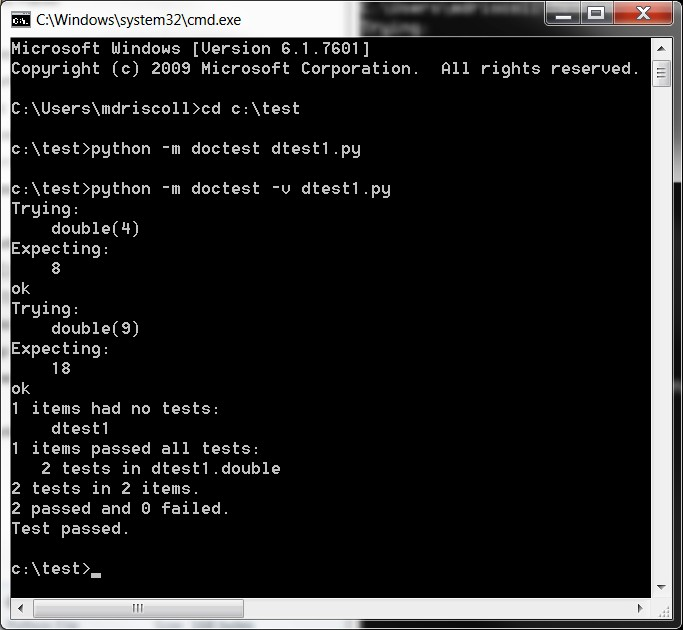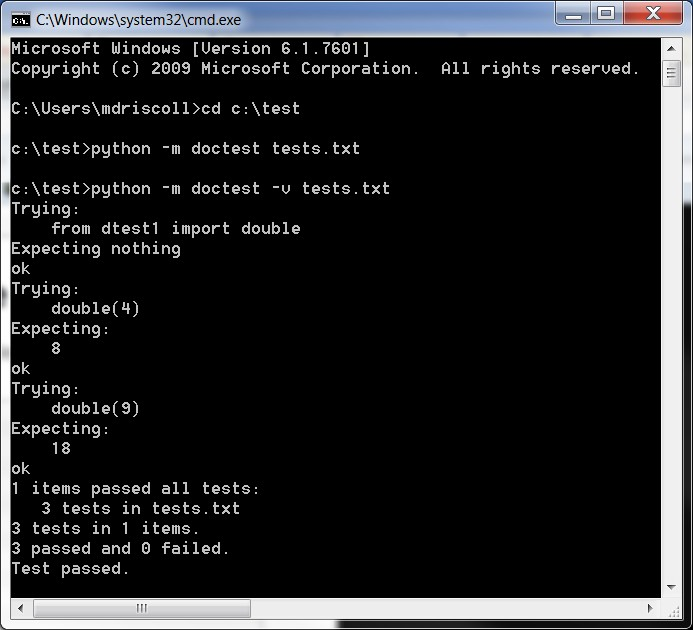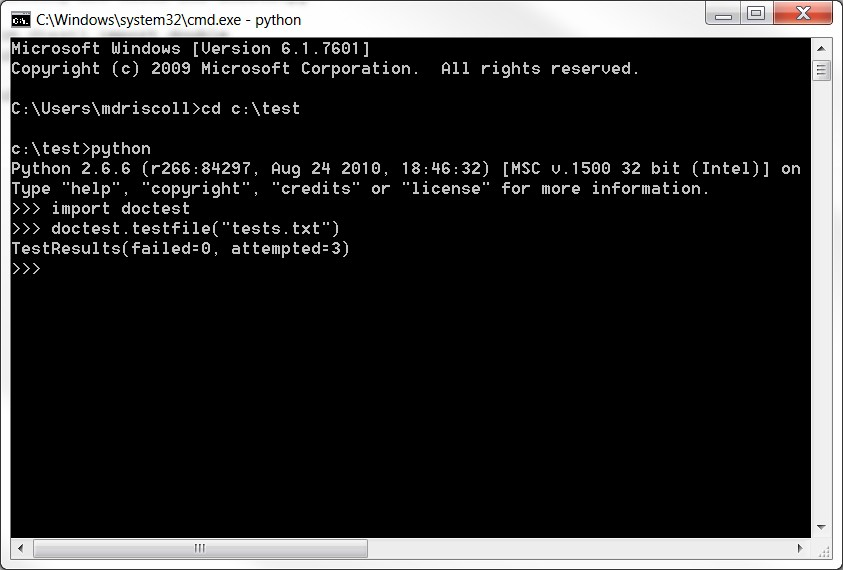# 第28章-测试简介¶

python包含两个用于测试代码的内置模块。这两种方法被称为 doctest单元测试 .我们来看看怎么用 doctest 首先，在第二部分中，我们将介绍使用测试驱动开发技术的单元测试。

## 用doctest测试¶

doctest模块将在代码中搜索类似于交互式Python会话的文本片段。然后，它将执行这些会话以验证它们的工作方式是否与所写的完全一致。这意味着，如果在显示带有尾随空格或制表符的输出的docstring中编写了一个示例，那么函数的实际输出也必须具有尾随空格。大多数情况下，docstring是您想要放置测试的地方。将涵盖doctest的以下方面：

• 如何从终端运行doctest

• 如何在模块内使用doctest

• 如何从单独的文件运行doctest

## 通过终端运行doctest¶

```# dtest1.py

def double(a):
"""
>>> double(4)
8
>>> double(9)
18
"""
return a*2
``````python -m doctest dtest1.py
```

```python -m doctest -v dtest1.py
```

“-v”意味着我们需要详细的输出，这正是我们收到的结果。再次打开代码，在docstring中的“18”后面添加一个空格。然后重新运行测试。这是我收到的输出：## 在模块内运行doctest¶

```def double(a):
"""
>>> double(4)
8
>>> double(9)
18
"""
return a*2

if __name__ == "__main__":
import doctest
doctest.testmod(verbose=True)
```

```python dtest2.py -v
```

## 从单独的文件运行doctest¶

doctest模块还支持将测试放入单独的文件中。这允许我们将测试与代码分开。让我们从上一个示例中剥离测试，并将它们放入名为 tests.txt

```The following are tests for dtest2.py

>>> from dtest2 import double
>>> double(4)
8
>>> double(9)
18
```## 第一次测试¶

```import unittest

class TestBowling(unittest.TestCase):
""""""

def test_all_ones(self):
"""Constructor"""
game = Game()
game.roll(11, 1)
self.assertEqual(game.score, 11)
```

```class Game:
""""""

def __init__(self):
"""Constructor"""
self.score = 0

def roll(self, numOfRolls, pins):
""""""
for roll in numOfRolls:
self.score += pins
```

```if __name__ == '__main__':
unittest.main()
```

```E
======================================================================
ERROR: test_all_ones (__main__.TestBowling)
Constructor
----------------------------------------------------------------------
Traceback (most recent call last):
File "C:\Users\Mike\Documents\Scripts\Testing\bowling\test_one.py",
line 27, in test_all_ones
game.roll(11, 1)
File "C:\Users\Mike\Documents\Scripts\Testing\bowling\test_one.py",
line 15, in roll
for roll in numOfRolls:
TypeError: 'int' object is not iterable

----------------------------------------------------------------------
Ran 1 test in 0.001s

FAILED (errors=1)
```

```def roll(self, numOfRolls, pins):
""""""
for roll in range(numOfRolls):
self.score += pins
```

```.
----------------------------------------------------------------------
Ran 1 test in 0.000s

OK
```

## 第二次测试¶

```from game import Game
import unittest

class TestBowling(unittest.TestCase):
""""""

def test_all_ones(self):
"""Constructor"""
game = Game()
pins = [1 for i in range(11)]
game.roll(11, pins)
self.assertEqual(game.score, 11)

def test_strike(self):
"""
A strike is 10 + the value of the next two rolls. So in this case
the first frame will be 10+5+4 or 19 and the second will be
5+4. The total score would be 19+9 or 28.
"""
game = Game()
game.roll(11, [10, 5, 4])
self.assertEqual(game.score, 28)

if __name__ == '__main__':
unittest.main()
```

```class Game:
""""""

def __init__(self):
"""Constructor"""
self.score = 0
self.pins = [0 for i in range(11)]

def roll(self, numOfRolls, pins):
""""""
x = 0
for pin in pins:
self.pins[x] = pin
x += 1
x = 0
for roll in range(numOfRolls):
if self.pins[x] == 10:
self.score = self.pins[x] + self.pins[x+1] + self.pins[x+2]
else:
self.score += self.pins[x]
x += 1
print(self.score)
```

## 第三次（也是最后一次）测试¶

```from game_v2 import Game
import unittest

class TestBowling(unittest.TestCase):
""""""

def setUp(self):
""""""
self.game = Game()

def test_all_ones(self):
"""
If you don't get a strike or a spare, then you just add up the
face value of the frame. In this case, each frame is worth
one point, so the total is eleven.
"""
pins = [1 for i in range(11)]
self.game.roll(11, pins)
self.assertEqual(self.game.score, 11)

def test_spare(self):
"""
A spare is worth 10, plus the value of your next roll. So in this
case, the first frame will be 5+5+5 or 15 and the second will be
5+4 or 9. The total is 15+9, which equals 24,
"""
self.game.roll(11, [5, 5, 5, 4])
self.assertEqual(self.game.score, 24)

def test_strike(self):
"""
A strike is 10 + the value of the next two rolls. So in this case
the first frame will be 10+5+4 or 19 and the second will be
5+4. The total score would be 19+9 or 28.
"""
self.game.roll(11, [10, 5, 4])
self.assertEqual(self.game.score, 28)

if __name__ == '__main__':
unittest.main()
```

```# game_v2.py

class Game:
""""""

def __init__(self):
"""Constructor"""
self.score = 0
self.pins = [0 for i in range(11)]

def roll(self, numOfRolls, pins):
""""""
x = 0
for pin in pins:
self.pins[x] = pin
x += 1
x = 0
spare_begin = 0
spare_end = 2
for roll in range(numOfRolls):
spare = sum(self.pins[spare_begin:spare_end])
if self.pins[x] == 10:
self.score = self.pins[x] + self.pins[x+1] + self.pins[x+2]
elif spare == 10:
self.score = spare + self.pins[x+2]
x += 1
else:
self.score += self.pins[x]
x += 1
if x == 11:
break
spare_begin += 2
spare_end += 2
print(self.score)
```